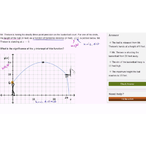# Class 11 Physics (India): Graph interpretation word problem: basketball

Need more information? Get more details on the site of the provider.### Description

When a function models a real-world context, we can learn a lot about the context from the function's graph. In this video, we interpret the y-intercept of a graph that models a basketball free throw.

## Class 11 Physics (India)

Let's learn, practice, and master topics of class 11 physics (NCERT) starting with kinematics and then moving to dynamics with Newton's laws of motion, work, energy, and power. Let's then use these as the foundation to learn about centre of mass, rotational motion, gravitation, solids, fluids, thermodynamics, and oscillations and waves.

## Interpreting features of graphs

Interpret the graphs of functions in terms of the contexts that are modeled by the functions.

## Topic: Sc…

There are no frequently asked questions yet. If you have any more questions or need help, contact our customer service.

When a function models a real-world context, we can learn a lot about the context from the function's graph. In this video, we interpret the y-intercept of a graph that models a basketball free throw.

## Class 11 Physics (India)

Let's learn, practice, and master topics of class 11 physics (NCERT) starting with kinematics and then moving to dynamics with Newton's laws of motion, work, energy, and power. Let's then use these as the foundation to learn about centre of mass, rotational motion, gravitation, solids, fluids, thermodynamics, and oscillations and waves.

## Interpreting features of graphs

Interpret the graphs of functions in terms of the contexts that are modeled by the functions.

## Topic: Science

Learn about all the sciences, from physics, chemistry and biology, to cosmology and astronomy, across hundreds of videos, articles and practice questions.

There are no reviews yet.